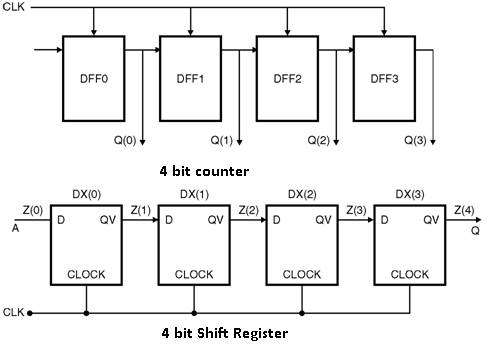Home > VHDL > Sequential Statements > If generate Statements

The if-generate statement is mainly used when we wanted to use generate as a concurrent statement. This is mainly needed when you are generating a series of repetitive statements or components and need to supply different parameters, or generate different components, at the beginning or end of the series. The syntax is :

if expression generate

{ concurrent_statement }

end generate ;

Example :

Ã˜ Example 1 : 4 bit counterlibrary ieee;

use ieee.std_logic_1164.all

entity counter4 is

port (COUNT,CLK: in std_logic;

Q : buffer std_logic_vector (3 downto 0));

end counter4;

architecture arch of counter4 is

component DFF is

port (D,CLOCK : in std_logic;

QV : out std_logic);

end component ;

begin

VK : for k in 0 to 3 generate

VK0 : if k = 0 generate

D1: DFF port map (COUNT,CLK,Q(k));

end generate VK0;

VK1_3:if k>0 generate

D1: DFF port map (Q(k-1),CLK,Q(k));

end generate VK1_3;

end generate VK;

end arch;

Ã˜ Example 2 : 4 bit shift register

library ieee;

use ieee.std_logic_1164.all

entity shift4 is

port (A,CLK: in std_logic;

Q: out std_logic);

end shift4;

architecture arch of shift4 is

component DFF is

port (D,CLOCK: in std_logic;

QV: out std_logic);

end component;

signal Z : std_logic_vector( 0 to 4);

begin

Z(0) <= A;

V 1: for k in 0 to 3 generate

DX : DFF port map (Z (k), CLK, Z (k+1));

end generate;

Q <=Z(4);

end arch;